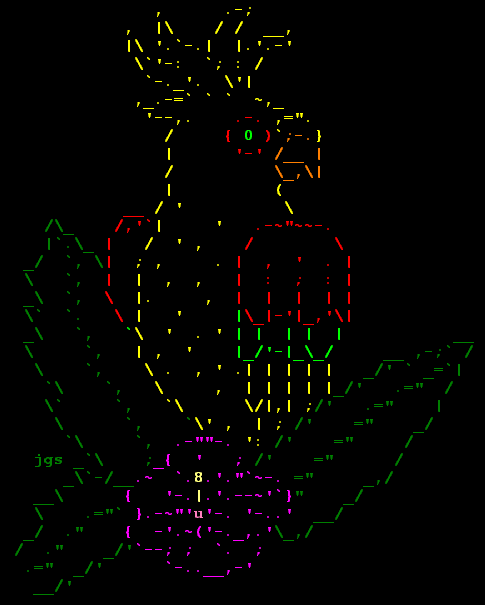# ASCII art

(redirected from Barphic)

## ASCII art

(graphics)
(Or "character graphics", "ASCII graphics") The fine art of drawing diagrams using the ASCII character set (mainly "|-/\+").

o----)||(--+--|<----+ +---------o + D O L )||( | | | C U A I )||( +-->|-+ | +-\/\/-+--o - T C N )||( | | | | P E )||( +-->|-+--)---+--)|--+-o U )||( | | | GND T o----)||(--+--|<----+----------+

A power supply consisting of a full wave rectifier circuit feeding a capacitor input filter circuit

Figure 1.

And here are some very silly examples:

|\/\/\/| ____/| ___ |\_/| ___ | | \ o.O| ACK! / \_ |` '| _/ | | =(_)= THPHTH! / \/ \/ | (o)(o) U / C _) (__) \/\/\/\ _____ /\/\/\/ | ,___| (oo) \/ \/ | / \/-------\ U (__) /____\ || | \ /---V `v'- oo ) / \ ||---W|| * * |--| || |`. |_/ //-o-\____---=======---____ ====___\ /.. ..\ /___==== Klingons rule OK! // ---\__O__/--- \\_\ /_/

_____ __...---'-----`---...__ _=============================== ,----------------._/' `---..._______...---' (_______________||_) . . ,--' / /.---' `/ '--------_- - - - - _/ `--------'

Figure 2.

There is an important subgenre of ASCII art that puns on the standard character names in the fashion of a rebus.

+--------------------------------------------------------+ | ^^^^^^^^^^^^ | | ^^^^^^^^^^^ ^^^^^^^^^ | | ^^^^^^^^^^^^^ ^^^^^^^^^^^^^ | | ^^^^^^^ B ^^^^^^^^^ | | ^^^^^^^^^ ^^^ ^^^^^^^^^^^^^^ | +--------------------------------------------------------+ "A Bee in the Carrot Patch"

Figure 3.

Within humorous ASCII art, there is, for some reason, an entire flourishing subgenre of pictures of silly cows. One is shown in Figure 2; here are three more:

(__) (__) (__) (\/) (\$\$) (**) /-------\/ /-------\/ /-------\/ / | 666 || / |=====|| / | || * ||----|| * ||----|| * ||----|| ~~ ~~ ~~ ~~ ~~ ~~ Satanic cow This cow is a Yuppie Cow in love

Figure 4.

http://gagme.wwa.com/~boba/scarecrow.html.
ASCII ARTAlthough simple, ASCII art can be very imaginative as in this example. (Image courtesy of Joan G. Stark.)